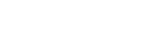### PATLITE Channel

Introducing You to Various Solutions

• ×

• ×

• ×

• ×

• ×

• ×

• ×

• ×

• ×

• ×

• ×

• ×

• ×

• ×

• ×

• ×

• ×

• ×

• ×

• ×

• ×

• ×

• ×

• ×

• ×

• ×

• ×

• ×

• ×

• ×

• ×

• ×

• ×

• ×

• ×

• ×

• ×

• ×

• ×

• ×

• ×

• ×

• ×

• ×

• ×

• ×

• ×

• ×

• ×

• ×

• ×

• ×

• ×

• ×

• ×

• ×

• ×

• ×

• ×

• ×

• ×

• ×

• ×

• ×

• ×

• ×

• ×

• ×

• ×

• ×

• ×

• ×

• ×

• ×

• ×

• ×

• ×

• ×

• ×

• ×

• ×

• ×

• ×

• ×

• ×

• ×

• ×

• ×

• ×

• ×

• ×

• ×

• ×

• ×

• ×

• ×

• ×

• ×

• ×

• ×

• ×

• ×

• ×

• ×

• ×

• ×

• ×

• ×

• ×

• ×